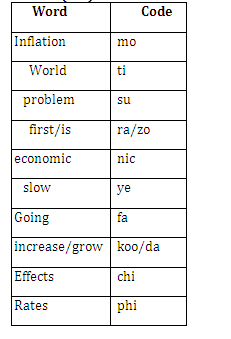# REASONING QUIZ FOR PUNJAB GOVT EXAMS 2020-21

Directions (Q1-Q5):Study the following information and answer the given questions.
In a certain code language,
‘world inflation is problem first’ is written as ‘suzotira mo’,
‘inflation effects economic’ is written as ‘chi monic’,
‘world economic going slow’ is written as ‘fanicti ye’,
‘increase rates slow grow’ is written as ‘phi ye koo da’
and ‘problem rates’ is written as ‘su phi’.

Q1.  What is the code for ‘first’?

A) su

B) zo

C) ra

D) Either zo or ra

E) None of these

Q2- What does ‘fa’ stand for?

A) world

B) slow

C) economic

D) going

E) Can’t be determined

Q3. Which of the following is the code for ‘problem rates effects grow’?

A) koo da phi chi

B) phisu nr chi

C)  ti da zo chi

D) su phi chi pa

E)  None of these

Q4.  Which of the following is represented by the code ‘mo ye su phi’?

A) economic is problem slow

B) inflation effects slow rates

C) problem inflation slow rates

D) slow economic grow rates

E) None of theseQ5.  Which of the following may be the code for ‘global is exceed tough problem first’?

A) niczosuramo pic

B) piczorasuvo bi

C) su pic ye zorafa

D) tirasu chi mozo

E) None of these.

Directions (6-10): Study the following information to answer the given questions.

In a certain code language,

‘last time the battle’ is written as ‘b%6i  g%4v  o#2c  s&3t’

‘time bat on final’ is written as ‘t%2m  o#2c  d#5a  p&3u’

‘on last kingdom calling’ is written as ‘n#6h  m%2k   t%2m  g%4v’ and

‘final king the on’ is written as ‘d#5a  s&3t  r%2x  t%2m’

Q6.In the given code language, what does the code ‘m%2k’ stand for?

(a) Either ‘last’ or ‘the’

(b) battle

(c) on

(d) Either ‘calling’ or ‘kingdom’

(e) king

Q7. What is the code for ‘final’ in the given code language?

(a) t%2m

(b) r%2x

(c) s#4t

(d) d#5a

(e) Other than those given as options

Q8. What is the code for ‘time’ in the given code language?

(a) n#6h

(b) o#2c

(c) g%4v

(d) p&3u

(e) r%2x

Q9. What is the code for ‘final bat’ in the given code language?

(a) v#6e be

(b) d#5a n#6h

(c) n#6h v&5e

(d) p&3u d#5a

(e) b%6i d#5a

Q10. If ‘on the home’ is written as ‘a\$4j t%2m s&3t’ in the given code language, then what is the code for ‘home bat battle’?

(a) a\$4j g%4v nh

(b) b%6i  p&3u   a\$4j

(c) b#6i  d#5a  a\$4j

(d) b%6i  d#5a  a\$4j

(e) b%6i  d%5a  a\$4j

## Solutions

Q (1 – 5) :Q1. Ans(D)

Q2. Ans(D)

Q3. Ans(E)

Q4. Ans(C)

Q5. Ans(B)

Q(6-10):

 Word Code Last g%4v Time o#2c The s&3t On t%2m Battle b%6i Final d#5a Bat p&3u King r%2x Kingdom/ calling m%2k/n#6h

Q6. Ans.(d)

Q7. Ans.(d)

Q8. Ans.(b)

Q9. Ans.(d)

Q10. Ans.(b)